# Solute Transport Phenomena with Input Through a Plane Surface in Porous Media

Solute Transport Phenomena with Input Through a Plane Surface in Porous Media
Department of Mathematics & Astronomy, University of Lucknow, Lucknow-226007, India

Corresponding Author Email:
Page:
557-565
|
DOI:
https://doi.org/10.18280/mmep.060411
12 September 2019
|
Accepted:
6 December 2019
|
Published:
24 December 2019
| Citation

OPEN ACCESS

Abstract:

In the present study, analytical solutions for conservative solute transport, originating from a plane input source, along and against the flow is studied in form of two cases through a three-dimensional semi-infinite porous media which is bounded between two infinitely spread planes. Also, flow is considered from one plane to another. In first case, concentration gradient perpendicular to the plane which is free from input is considered zero i.e. $\left(D_{h} \cdot \nabla c\right) \cdot \hat{n}=0$ while in second case concentration gradient perpendicular to the plane which is free from input is assumed to be proportional to concentration at that plane i.e. $\left(D_{h} \cdot \nabla c\right) \cdot \hat{n} \propto c$. Here $D_{h}$ is second order tensor represents dispersion coefficient and $\hat{n}$ is unit normal vector to the corresponding plane. In both cases, initially the aquifer is supposed to be uniformly polluted. The temporarily dependent dispersion is assumed proportional to the groundwater velocity. The analytical solution of the advection-dispersion equation is obtained using Laplace Transformation Technique. A transformation is used to change the time-dependent advection-dispersion equation into constant coefficients. Such results may be very useful in predicting the concentration pattern in real scenario where the solute material is injected through a plane in a medium. The prosed model may be used to predict concentration profiles of solute in the laboratory as well as in the field.

Keywords:

advection, dispersion, porous medium, groundwater velocity, Laplace transformation technique

1. Introduction

Contamination of groundwater by excessive use of pesticides in agriculture, unplanned storage of municipal garbage and unplanned urbanization is a major threat to environment. There are several aspects of solute transport in subsurface even in surface that still be poorly addressed by researchers. Mathematical modelling is the best tool to predict the solute transport in groundwater and in the assessment of its effect to human health and environment.

Advection-dispersion equation is commonly used to describe solute transport in an aquifer due to combine effect of diffusion and dispersion. Dispersion relate to the dissemination of contaminants in aquifers is dominated by the special structure of the geological formation. The mathematical studies suggest that the dispersion coefficients vary temporally and specially due to inhomogeneity of the medium.

Human made system may be helpful for illustrating the solution for temporal dependent input function. Majority of analytical solutions available in the literatures are either for constant velocity and dispersion coefficient or unsteady velocity and variable dispersion coefficient with different boundary conditions. Fried  and Rumer  derived longitudinal dispersion coefficient for one-dimensional flow experimentally within a certain range of Reynolds numbers. In geological formations, dispersivity may change with position or time for uniform flow in large subsurface Matheron and Marsily . Huang et al.  developed an analytical solution for one-dimensional solute transport in heterogeneous geological formation with a scale-dependent dispersion. Singh et al.  studied the solute transport phenomenon with time dependent velocities of sinusoidal, asymptotic and exponentially decreasing natures. Time dependent input function plays a crucial role in description contaminant transport through a natural system in which the inlet at a boundary is some function of time Logan , Zlotnik  and Ledder . Ellsworth and Butters  employed Green’s function for solute transport with arbitrary boundary conditions. Batu  provided two-dimensional analytical solution for solute transport in bounded aquifer using Fourier analysis and Laplace transform Technique. Aral and Liao  developed two-dimensional analytical solutions for temporarily dependent dispersion coefficients in an infinite porous domain. Zoppou and Knight  presented analytical solutions for advection-diffusion equations for conservative solute dispersion being proportional to the square of the velocity and position variable. Van Genuchten et al.  developed one and multi-dimensional solutions for solute transport with and without terms accounting for zero-order production and first-order decay. Mahato et al.  obtained analytical solution for two-dimensional solute transport problem in a saturated porous media. Previous studies ordinarily considered solute transport subject to a different type of temporarily dependent inlet boundary conditions. Batu , Batu  have studied the two-dimensional analytical solutions for plane sources with the first and second type boundary conditions. Park and Zhan  derived general form of analytical solutions of solute transport from three-dimensional sources in a finite thickness aquifer using Green Function Method. Zamani and Bombardelli  proposed analytical solutions of nonlinear advection-dispersion equation with spatially and temporally dependent dispersion coefficient and velocity. Kumar et al.  obtained solution for a two-dimensional solute transport problem with spatially varying dispersion and groundwater velocity. Yadav and Roy  studied solute transport phenomena with curved line and curved surface sources in two- and three- dimensional semi-infinite porous media respectively by using Laplace Transformation Technique. Thakur et al.  obtained exact solutions for two-dimensional solute transport with unsteady dispersion and groundwater velocity in homogeneous and heterogeneous porous media and compared the concentration distribution patterns in the considered media. Pandey et al.  explored solute transport phenomena in a heterogeneous porous media with Dirichlet type boundary condition by obtaining the solution using Duhamel’s Principle.

To the best of our knowledge; currently very few analytical solutions to advection-dispersion equation with a plane surface are available for demonstrating temporally dependent solute transport problems. The objective of this study is to extend the work of Singh and Chatterjee  and derive analytical solution to the advection-dispersion equation with a constant uniform plane input source for conservative solute. Constant uniform plane input sources applied in direction and against the flow for a porous medium lying between two parallel planes of infinite length are studied in separate cases. Flow velocity is time dependent and dispersion is considered proportional to flow velocity. A mathematical formulation of the solute transport problem is presented in two sections to explore the impact of transport parameters within the porous domain. As particular cases, solution for line and point source in one and two-dimensional advection-dispersion equation are also discussed. The effects of the colony of dispersion with time and the other parameters of the porous domain on the solute transport are studied and illustrated separately with the help of graphs.

2. Mathematical Formulation and Solution of Problem

The general geometry of the problems considered in this study can be described as shown Figure 1 (a) & Figure (b). The aquifer is assumed to be of semi-infinite length in the x, y and z directions. The aquifer is considered without curvature. The groundwater flow transporting the solute is considered to have components along the x, y, z axes. Temporal distribution of groundwater flow that enters the domain mainly affects the solute migration. We consider the transport of a contaminant through a homogeneous semi-finite aquifer bounded between two infinitely long planes. Direction of flow is assumed from left plane to right plane. Input is applied at one plane while second boundary condition is considered at other plane i.e., present article deals with two cases namely (1) input in the direction and (2) against the flow.

Initially, it is assumed that the porous domain is uniformly polluted for both the cases. The governing equation of solute transport can be expressed by three-dimensional advection-dispersion equation (ADE) Bear , Yadav et al.  as follows:

$R \frac{\partial c}{\partial t}=\frac{\partial}{\partial x}\left\{D_{x x}(x, y, z, t) \frac{\partial c}{\partial x}-u_{x}(x, y, z, t) c\right\}+$$\frac{\partial}{\partial y}\left\{D_{y y}(x, y, z, t) \frac{\partial c}{\partial y}-u_{y}(x, y, z, t) c\right\}+$$\frac{\partial}{\partial z}\left\{D_{z z}(x, y, z, t) \frac{\partial c}{\partial z}-u_{z}(x, y, z, t) c\right\}$        (1)

where, $c(x, y, z, t)\left[M L^{-3}\right]$ is concentration with position $x[L], y[L], z[L]$ and at time $t[T]$ in liquid phase, $D_{x x}\left[L^{2} T^{-1}\right], \quad D_{y y}\left[L^{2} T^{-1}\right]$ and $D_{zz}\left[L^{2} T^{-1}\right]$ are components of dispersion coefficients while $u_{x}\left[L T^{-1}\right], u_{y}\left[L T^{-1}\right]$ and $u_{z}\left[L T^{-1}\right]$ are components of groundwater velocity of the medium in direction of coordinate axes x, y and z respectively. R is the retardation factor which is a dimensionless quantity. It should be noted that retardation process may also happen when solute enters the porous domain.

## 1a.jpg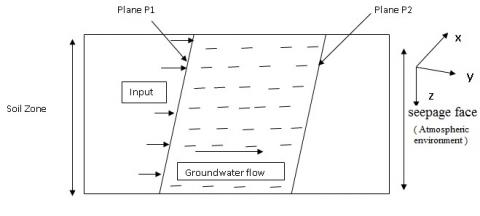(a) Cross section of the Modeled System for along the flow input

## 1b.jpg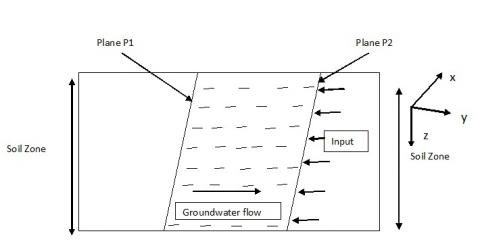(b) Cross section of the Modeled System for against the flow input

Figure 1. Geometry of plane source contamination

Dispersion coefficient and velocity along x, y and z directions are considered as follows:

$\left.\begin{array}{rl}{D_{xx}}{=D_{x x 0} f(m t); D_{y y}=D_{y y 0} f(m t) ; D_{z z}=D_{zz 0} f(m t)} \\ {u_{x}}{=u_{x 0} f(m t) ; u_{y}=u_{y 0} f(m t) ; u_{z}=u_{z 0} f(m t)}\end{array}\right\}$        (2)

where, $D_{\mathrm{xx0}}, D_{\mathrm{yy} 0}, D_{\mathrm{zz} 0}$ and $u_{x 0}, \quad u_{y 0}, \quad u_{z 0}$ are constants. m is an unsteady parameter having dimension inverse of time. Function $f(m t)$ defines time dependency of dispersion and velocity components. The obtained results are also discussed for two sub cases namely line and point source.

2.1 Case 1. Input in direction the flow

The geometry of the present case is shown in Figure 1(a). The initial and boundary conditions considered herein may be given as:

$c(x, y, z, t)=c_{i} ; f_{1} \leq a_{1} x+b_{1} y+e_{1} z \leq f_{2}, t=0$   (3)

$c(x, y, z, t)=c_{0} ; a_{1} x+b_{1} y+e_{1} z=f_{1}, t>0$     (4)

$\left(D_{h}. \nabla c\right).\hat{n}=0$ at $a_{1} x+b_{1} y+e_{1} z=f_{2}, t \geq 0$   (5a)

where, $\hat{n}$ is normal to the plane P2. The Eq. (5a) takes the following form (pp 251 Bear , pp71 Bear and Verruijt ).

$D_{x x 0} a_{1} \frac{\partial c}{\partial x}+D_{y y 0} b_{1} \frac{\partial c}{\partial y}+D_{zz 0} e_{1} \frac{\partial c}{\partial z}=0 ;$ at $a_{1} x+b_{1} y+e_{1} z=f_{2}$,$t \geq 0$       (5b)

The boundary condition Eq. (5a) and (5b) in three-dimensional flow is corresponding to Case II condition of Jaiswal et al.  in one-dimensional flow. i.e. taking $D_{y y 0}=D_{zz 0}=0$ and $u_{y 0}=u_{z 0}=0$ in Eq.(5b) it reduces to condition of boundary condition against the flow Jaiswal et al. .

where, $c_{i}, c_{0}$ are initial and reference concentrations. Also $a_{1}(\neq 0)[L], b_{1}[L], e_{1}[L]$ and $f_{1}, f_{2} \quad\left(f_{1}<f_{2}\right)$ are real numbers. $f_{1}, f_{2}$ are dimensionless. Throughout the study we denote plane $a_{1} x+b_{1} y+e_{1} z=f_{1}$ and $a_{1} x+b_{1} y+e_{1} z=f_{2}$ by P1 and P2 plane respectively. $D_{h}$ is second order tensor represents dispersion coefficient where $D_{i j},$ such that $i, j=x, y, z$ $\left(D_{i j}=0 \text { when } i \neq j\right.$ and $\left.D_{x x}=D_{x x 0}, D_{y y}=D_{y y 0}, D_{z z}=D_{zz 0}\right)$ is called principal axis of dispersion [22, 24]. Eq. (5a, 5b) represents boundary of exit to the atmosphere The Seepage flow may serve as an example . Introducing a new time variable T to reduce the Eq. (1) into constant coefficient. The variable T may be defined as Crank :

$T=\int_{0}^{t} f(m t) d t$    (6)

With this time variable T, Eq. (1) and Eqns. (3-5) convert into following form:

$R \frac{\partial c}{\partial T}=\left\{D_{x x 0} \frac{\partial^{2} c}{\partial x^{2}}-u_{x 0} \frac{\partial c}{\partial x}\right\}+\left\{D_{y y 0} \frac{\partial^{2} c}{\partial y^{2}}-u_{y 0} \frac{\partial c}{\partial y}\right\}+$$\left\{D_{z z 0} \frac{\partial^{2} c}{\partial z^{2}}-u_{z 0} \frac{\partial c}{\partial z}\right\} (7) c(x, y, z, T)=c_{i} ; f_{1} \leq a_{1} x+b_{1} y+e_{1} z \leq f_{2}, T=0 (8) c(x, y, z, T)=c_{0} ; a_{1} x+b_{1} y+e_{1} z=f_{1}, T>0 (9) D_{x x 0} a_{1} \frac{\partial c}{\partial x}+D_{y y 0} b_{1} \frac{\partial c}{\partial y}+D_{zz 0} e_{1} \frac{\partial c}{\partial z}=0 ; at a_{1} x+b_{1} y+e_{1} z=f_{2},T \geq 0 (10) In order to reduce the Eq. (7) into one-dimensional or single space variable, we introduce a new transformation as Singh and Chatterjee : Z=x+\frac{b_{1}}{a_{1}} y+\frac{e_{1}}{a_{1}} z-\frac{f_{1}}{a_{1}} (11) Using transformation Eq. (11), Eqns. (7-10) takes following form: R \frac{\partial c}{\partial T}=D \frac{\partial^{2} c}{\partial Z^{2}}-U \frac{\partial c}{\partial Z} (12) where, D=D_{xx0}+\frac{b_{1}^{2}}{a_{1}^{2}} D_{yy 0}+\frac{e_{1}^{2}}{a_{1}^{2}} D_{zz 0} and U=u_{x_{0}}+\frac{b_{1}}{a_{1}} u_{y_{0}}+\frac{e_{1}}{a_{1}} u_{z_{0}} c(Z, T)=c_{i} ; 0 \leq Z \leq L, T=0 (13) c(Z, T)=c_{0} ; Z=0, T>0 (14) \frac{\partial c}{\partial Z}=0 ; \quad as \quad Z=L, T \geq 0 (15) where, L=\frac{f_{2}-f_{1}}{a_{1}}. We further use following transformation to eliminate convective part of Eq. (12) c(Z, T)=k(Z, T) \exp \left[\frac{U}{2 D} Z-\frac{U^{2}}{4 R D} T\right] (16) Eqns. (12-15) reduce into following form: R \frac{\partial k}{\partial T}=D \frac{\partial^{2} k}{\partial Z^{2}} (17) k(Z, T)=c_{i} \exp (-\beta Z) ; 0 \leq Z \leq L, T=0 (18) k(Z, T)=c_{0} \exp \left[\eta^{2} T\right] ; Z=0, T>0 (19) \frac{\partial k}{\partial Z}+\frac{U}{2 D} k=0 ; at Z=L, T \geq 0 (20) where, \eta=\sqrt{\frac{U^{2}}{4 R D}} and \beta=\frac{U}{2 D}. Applying Laplace Transform Technique to Eqns. (17-20), one can get the solution of the present problem. For this purpose, we take Laplace Transform of Eqns. (17-20). \frac{d^{2} \bar{k}}{d Z^{2}}-\frac{p R}{D} \bar{k}=-\frac{R}{D}\left\{c_{i} \exp (-\beta Z)\right\} (21) \bar{k}(Z, p)=\frac{c_{0}}{\left(p-\eta^{2}\right)} ; Z=0 (22) \frac{d \bar{k}}{d Z}+\frac{U}{2 D} \bar{k}=0 ; at Z=L (23) General solution of Eq. (21) may be written as: \bar{k}(Z, p)=C_{1} \cosh (M Z)+C_{2} \sinh (M Z)+\frac{c_{i} \exp (-\beta Z)}{\left(p-\rho^{2}\right)} (24) Using Eqns. (21, 22) along with Eq. (23), we get \bar{k}(Z, p)=\left\{\frac{c_{0}}{\left(p-\eta^{2}\right)}-\frac{c_{i}}{\left(p-\rho^{2}\right)}\right\}$$\frac{M \cosh \{M(Z-L)\}+\beta \sinh \{M(L-Z)\}}{M \cosh (M L)+\beta \sinh (M L)}+$$\frac{c_{i} \exp (-\beta Z)}{\left(p-\rho^{2}\right)} (25) where, M=\sqrt{\frac{p R}{D}} and \rho=\sqrt{\frac{D}{R} \beta^{2}}. Taking inverse Laplace transform of Eq. (25) and then using Eq. (16), solution of present case may be written as Jaiswal et al. : c(Z, T)=\left[c_{0}\left\{E_{1} \exp \left(\eta^{2} T\right)-2 D L \sum_{n=1}^{\infty} \omega_{n}^{2} E_{21} \exp \left(-\frac{\omega_{n}^{2} D}{R L^{2}} T\right)\right\}-\right.$$c_{i}\left\{E_{3} \exp \left(\rho^{2} T\right)-2 D L \sum_{n=1}^{\infty} \omega_{n}^{2} E_{23} \exp \left(-\frac{\omega_{n}^{2} D}{R L^{2}} T\right)\right\}+$$\left.c_{i} \exp \left(\rho^{2} T-\beta Z\right)\right] \times \exp \left[\frac{U}{2 D} Z-\frac{U^{2}}{4 R D} T\right] (26) where, E_{1}=\frac{G_{1}}{G_{2}} ; E_{21}=\frac{G_{3}}{G_{4}} ; E_{3}=\frac{G_{5}}{G_{6}} ; E_{23}=\frac{G_{3}}{G_{7}} and, G_{1}=\gamma \cosh \{\gamma(L-Z)\}+\beta \sinh \{\gamma(L-Z)\}, G_{2}=\gamma \cosh (\gamma L)+\beta \sinh (\gamma L), G_{3}=\frac{\omega_{n}}{L} \cosh \left\{\frac{\omega_{n}(L-Z)}{L}\right\}+\beta \sinh \left\{\frac{\omega_{n}(L-Z)}{L}\right\}, G_{4}=\left(\omega_{n}^{2} D+\eta^{2} R L^{2}\right)\left\{\left(\omega_{n}^{2}+\beta^{2} L^{2}\right)+\beta L\right\} \sin \left(\omega_{n}\right), G_{5}=\varphi \cosh \{\varphi(L-Z)\}+\beta \sinh \{\varphi(L-Z)\}, G_{6}=\varphi \cosh (\varphi L)+\beta \sinh (\varphi L), G_{7}=\left(\omega_{n}^{2} D+\rho^{2} R L^{2}\right)\left\{\left(\omega_{n}^{2}+\beta^{2} L^{2}\right)+\beta L\right\} \sin \left(\omega_{n}\right) where, \omega_{n} is the positive root of \omega_{n} \cot \left(\omega_{n}\right)+\beta L=0. Also, \gamma=\eta \sqrt{R / D} and \varphi=\rho \sqrt{R / D}. 2.2 Case2. Input in against the flow The geometry of the present case of the problem is elaborated in Figure 1(b). In the present study, solute transport originating from a plane source P2 against the directions of groundwater flow keeping the geometry of aquifer unaltered. Present case may be represented mathematically by replacing Eqns. (4, 5a, b) with Eqns. (27a, b and 28) as: \left(D_{h} . \nabla c\right) \cdot \hat{n}=\frac{1}{2}\left({\bar{u} \cdot \hat{n}}\right) c at a_{1} x+b_{1} y+e_{1} z=f_{1}, \quad t \geq 0 (27a) where, \hat{n} is normal to the plane P1. The Eq. (27a) takes the following form: D_{x x 0} \frac{\partial c}{\partial x}+D_{y y 0} \frac{b_{1}}{a_{1}} \frac{\partial c}{\partial y}+D_{z z 0} \frac{e_{1}}{a_{1}} \frac{\partial c}{\partial z}=\frac{U}{2} c at a_{1} x+b_{1} y+e_{1} z=f_{1}, t \geq 0 (27b) c(x, y, z, t)=c_{0} ; a_{1} x+b_{1} y+e_{1} z=f_{2}, t>0 (28) Initial condition is taken similar to previous case i.e. Eq. (3). The boundary condition Eq. (27) in three-dimensional flow is corresponding to Case I condition Jaiswal et al.  in one dimensional flow. i.e. taking D_{y y 0}=D_{zz0}=0 and u_{y 0}=u_{z 0}=0 in Eq. (27b) it reduces to condition of boundary condition against the flow Jaiswal et al , Yadav et al. , Yadav et al. . Applying transformations in Eqns. (6, 11, 16), Eqns. (27b, 28) convert into following form: \frac{\partial k}{\partial Z}=0 ; \quad at Z=0, T>0 (29) k(Z, T)=c_{0} \exp (-\beta L) \exp \left[\eta^{2} T\right] ; Z=L, T>0 (30) where, \eta=\sqrt{\frac{U^{2}}{4 R D}} and \beta=\frac{U}{2 D}. Taking Laplace Transformation of Eqns. (29, 30), we get, \frac{d \bar{k}}{d Z}=0 at Z=0 (31) \bar{k}(Z, p)=\frac{c_{0} \exp (-\beta L)}{\left(p-\eta^{2}\right)} ; Z=L (32) Using above conditions Eq. (31, 32), the solution of Eq. (24) may be written as: \bar{k}(Z, p)=\left\{\frac{c_{0}}{\left(p-\eta^{2}\right)}-\frac{c_{i}}{\left(p-\rho^{2}\right)}\right\} \exp (-\beta L) \frac{\cosh (M Z)}{\cosh (M L)}$$-\frac{c_{i} \beta}{\left(p-\rho^{2}\right)} \frac{\sinh [M(L-Z)]}{M \cosh (M L)}+\frac{c_{i} \exp (-\beta Z)}{\left(p-\rho^{2}\right)}$  (33)

where, $M=\sqrt{\frac{p R}{R}}$ and $\rho=\sqrt{\frac{D}{R} \beta^{2}}$.

Taking Inverse Laplace Transform of Eq. (33) and then using Eq. (16), the desired solution may be written as Jaiswal et al. , Yadav et al. , Yadav et al. :

$c(Z, T)=\left[c_{0} \exp (-\beta L)\left\{E_{\eta} \exp \left(\eta^{2} T\right)-2 \pi D L \sum_{n=1}^{\infty} E_{1} \exp \left(-\frac{\omega_{n}^{2}}{L^{2}} D T\right)\right\}-\right.$$c_{i} \exp (-\beta L)\left\{E_{\rho} \exp \left(\rho^{2} T\right)-2 D \pi L \times \sum_{n=1}^{\infty} E_{2} \exp \left(-\frac{\omega_{n}^{2}}{L^{2}} D T\right)\right\}- c_{i}(-\beta)\left\{H_{\rho} \exp \left(\rho^{2} T\right)-2 D L \sum_{n=1}^{\infty} H_{1} \exp \left(-\frac{\omega_{n}^{2}}{L^{2}} D T\right)\right\}+$$\left.c_{i} \exp \left(\rho^{2} T-\beta Z\right)\right] \times \exp \left[\frac{U}{2 D} Z-\frac{U^{2}}{4 R D} T\right]$  (34)

where,

$E_{\sigma}=\frac{\cosh [\sigma \sqrt{R / D} Z]}{\cosh (\sigma \sqrt{R / D} L)} ; E_{1}=\frac{G_{1}}{G_{\eta}} ; E_{2}=\frac{G_{1}}{G_{\rho}}$

$H_{\sigma}=\frac{\sinh [\sigma \sqrt{R / D}(Z-L)]}{(\sigma \sqrt{R / D}) \cosh (\sigma \sqrt{R / D} L)} ; H_{1}=\frac{I_{1}}{I_{\rho}}$

and

$G_{1}=(-1)^{n}\left(n+\frac{1}{2}\right) \cos \left\{\left(n+\frac{1}{2}\right) \pi\left(\frac{Z}{L}\right)\right\}$;

$G_{\sigma}=\left\{D^{2} \pi^{2}\left(n+\frac{1}{2}\right)^{2}+\sigma^{2} R L^{2}\right\}$;

$I_{1}=(-1)^{n} \sin \left\{\left(n+\frac{1}{2}\right) \pi\left(\frac{Z-L}{L}\right)\right\}$;

$I_{\sigma}=\left\{D^{2} \pi^{2}\left(n+\frac{1}{2}\right)^{2}+\sigma^{2} R L^{2}\right\}$;

Also $\gamma=\eta \sqrt{R / D}$ and $\varphi=\rho \sqrt{R / D}$, where, $\omega_{n}$ is the positive root of $\cos \left(\omega_{n}\right)=0$ i.e. $\omega_{n}=\left(n+\frac{1}{2}\right) \pi$.

Parallel plane concept is used to obtain the closed-form solution for various dispersion functions including line source in two-dimension and   point source in one-dimension as special cases.

Now considering $D_{z z 0}=0, u_{z_{0}}=0$ and $e_{1}=0$ in Eq. (26, 34), we obtained solution of two-dimensional ADE for a line source in the direction of the flow in an infinite porous domain bounded between two infinite long lines line L1: $a_{1} x+b_{1} y=f_{1}$ and line L2: $a_{1} x+b_{1} y=f_{2}$ for along and against the flow.

Similarly, Putting, $D_{y y 0}=D_{zz=0}=0 \quad, u_{y_{0}}=u_{z_{0}}=0, a_{1}=1$, $b_{1}=0, e_{1}=0$ and $f_{1}=0$ in  Eq.(26, 34), solution of one-dimensional ADE for point source in a finite length of  porous domain for along and against the flow.

3. Result and Discussion

Various scenarios are considered to demonstrate the solute migration with temporarily dependent dispersion coefficient. In all scenarios, solute migration along and against the flow with a constant uniform input plane sources are considered. In order to present several graphical illustrations, numerical values of parameters, which are considered on the basis of some available literatures, are given as: Initially, aquifer is considered uniformly polluted with concentration $c_{i}=10 \mathrm{mg} / l$. Yadav et al. . The source plane is considered at concentration $c_{0}=1000 \mathrm{mg} / l$ in both considered cases Singh and Chatterjee . Dispersion coefficient and groundwater velocity in medium are considered exponentially decreasing function of time i.e., $f(m t)=\exp (-m t)$ with value of unsteady parameters is taken $m\left(y e a r^{-1}\right)=0.1$. The components of groundwater velocity along x, y and z axes directions are respectively $u_{x_{0}}=0.0121\left({km} { year }^{-1}\right)$, $u_{y_{0}}=0.030\left(k m \quad y e a r^{-1}\right)$ and $u_{z_{0}}=0.070\left(k m \quad y e a r^{-1}\right)$. The value of temporally dependent groundwater velocity is taken within the groundwater velocity range from 2m per day to 2m per year Todd . Further, the components of dispersion coefficients along x, y and z axes directions are respectively $D_{x x 0}=0.01\left(k m^{2} y e a r^{-1}\right)$, $D_{y y 0}=0.04\left(k m^{2} y e a r^{-1}\right), \quad D_{z z 0}=0.09\left(k m^{2} y e a r^{-1}\right)$. Analytical solutions given by Eqns. (26) and Eqns.(34) in the domain $f_{1} \leq a_{1} x+b_{1} y+e_{1} z \leq f_{2}$ for three dimensional porous medium lying between two infinite parallel planes described by $a_{1}=1 ; b_{1}=2 ; e_{1}=3 ; f_{1}=2.4$ and $f_{2}=3.4$.

3.1 Case1. Input in direction of flow

Figure 2 is drawn to understand the concentration distribution in Figure 3. From point P on plane P1 three lines PQ, PR and PS having direction cosines / ratios (d.r.) (l1,m1,n1), (l2,m2,n2) and (l3,m3,n3) respectively are shown where points Q, R and S lie on the Plane P2. Following Figure 3 exhibits concentration pattern along three directions mentioned in the figure.

The point represented in blue circle denotes level of concentration at point P (0.1,1.0,0.1) on the plane P1 and points in yellow are representing concentration level at points Q, R, S on plane P2. The direction ratios of PQ, PR and PS lines are (1,2,3), (3,2,1) and (1,3,2) respectively. Concentration pattern is evaluated along these mentioned directions PQ, PR and PS at times $t(\text { year })=0.08$ and 0.5. Since plates are of infinitely long, concentration distribution on planes at a particular time must be same. It may be observed that level of concentration is same at plane P2 irrespective of directions approached from point P on plane P1 to plane P2. Different values of components dispersion and groundwater velocity give rise to different concentration patterns in different directions from a point on plane P1 .Concentration at time $t(\text { year })=0.08$ and 0.5 are represented by solid and dashed line in all the three directions. Concentration level starts from constant on plane P1 and proceeds to its lowest level at plane P2. As time increases, contaminant concentration increases inside the domain.

## 2.png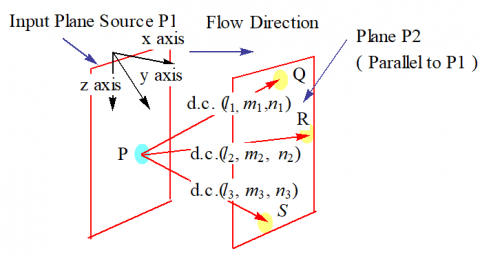Figure 2. Representing the method of graph plotting in figure 3

## 3.pngFigure 3. Concentration pattern obtained with Eq. (26) for plane source along three different directions at times t(year)=0.08, 0.5

Figure 4, Figure 5 and Figure 6 illustrate the solute concentration profiles along xy, yz and zxx plane of the medium starting from the point M (0.8, 0.8, 0) on plane P1, described by the solution in Eq. (26)  at time t(year)=0.08.

Concentration profile demonstrated in figure 4 in xy plane for a fixed z coordinate at z=0. The dimensionless concentrations c/c0 is constant at point (0.8, 0.8, 0) on source plane P1 and varies on moving way from this plane P1 for fixed z. Surface plot represents an exclusive pattern of concentration in observed plane. It may be noticed that the decreasing rate of concentration is faster along y axis in comparison to x axis from plane P1. This pattern of concentration is with similar plot done in Singh and Chatterji  at large extant. Concentration reduces to its lowest at point (1.13,1.13,0) on plane P2 at time t(year)=0.08.

The Figure 5 explores concentration distribution in yz plane for a fixed x coordinate at x=0.8. Since source plane P1 is at fixed concentration, the concentration injected through plane P1 disperses in the medium and reaches to plane P2. It is noticed that the concentration level decreases on moving away from point M (0.8, 0.8, 0) on the plane P1. On moving equal distance from point M along y and z axes, we observe concentration level drops faster along z axes in comparison to same along y axes. In yz plane concentration is recorded lowest at point (0.8, 1.13, 0.11) which is on plane P2.

## 4.jpg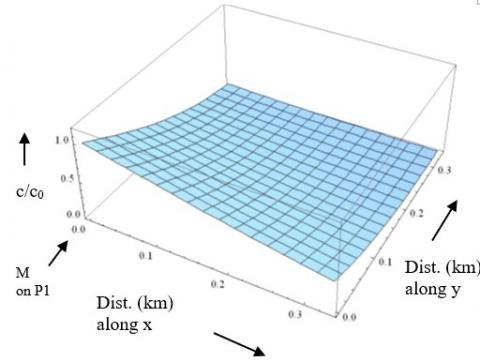Figure 4. Surface plot of concentration obtained with Eq. (26) for plane source at time t(year)=0.08 in xy plane

## 5.jpgFigure 5. Surface plot of concentration obtained with Eq. (26) for plane source at time t(year)=0.08 in yz plane

## 6.jpgFigure 6. Surface plot of concentration obtained with Eq. (26) for plane source at time t(year)=0.08 in xz plane

In present Figure 6, a surface plot demonstrates the  concentrations pattern in xz plane for a fixed y coordinate at y=0.8. The point (1.13,0.8,0.22) which is diagonally opposite to point M is on the plane P2 is at lowest level of concentration. The dimensionless concentration c/c0 is constant and equal to input concentration 1 at point (0.8,0.8,0) on source plane P1. Like pattern of Singh , concentration reduces more rapidly with distance along z axis in comparison to x axis.

3.2 Input in against the flow

Like Figure 2, the Figure 7 is plotted to demonstrate the concentration plotting of Figure 8 from point P on plane P1 along three lines PQ, PR and PS having direction ratios (l1,m1,n1) ,(l2,m2,n2) and (l3,m3,n3),where points Q, R and S lie on the Plane P2.  In Figure 8, concentration pattern along three mentioned direction is obtained for an input against the flow i.e. from plane P2 to P1.

## 7.png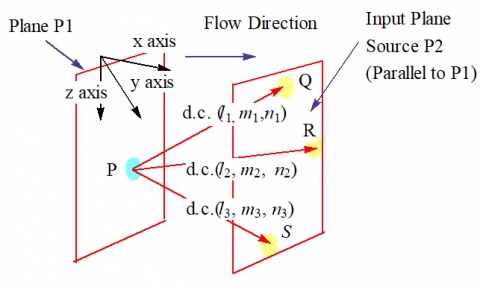Figure 7.  Representing the method of graph plotting in figure 8

## 8.png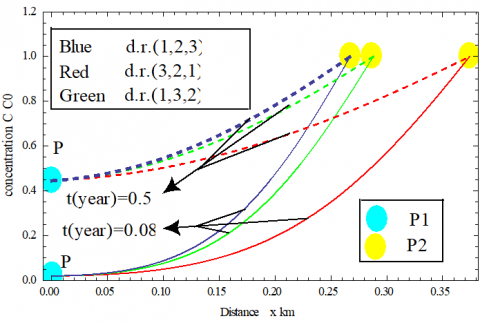Figure 8. Concentration pattern obtained with Eq. (34) for plane source along three different directions at times t(year)=0.08, 0.5

The points depicted in blue circle express the level of concentrations at point P (0.1,1.0,0.1) on the plane P1 while yellow circles are representing concentration levels at points Q, R, and S on plane P2. The positions of Q, R and S are defined same as it is defined in figure 3. Since solute is injected against the flow from Plane P2 concentrations levels at Q, R and S are constant i.e., $c / c_{0}=1$. Concentration decreases on moving from plane P2 to P1. Concentrations at time t(year)=0.08 and 0.5 are represented by solid and dashed line respectively in all the three directions. Similar to above case, the concentrations increase with time inside the domain. Concentration throughout the planes P1 and P2 is at same level at a particular time. Comparing the solution pattern at time t(year)=0.5 of present case with pattern corresponding figure of previous case. It is observed concentration level inside the domain as well as plane other than the input in against the flow is less in comparison to same of along the flow.

Surface plots in Figure 9, Figure 10 and Figure 11 give view of concentration distribution generated from Eq. (34) along the plane xy, yz and zx inside the medium starting from the point M(0.8,0.8,0) on plane P1, at time t(year)=0.08.

In Figure 9, concentration profile demonstrated in xy plane for a fixed z coordinate at z=0 with a surface plot. The dimensionless concentration $c / c_{0}$ is constant i.e., $c / c_{0}=1$ at point (1.13,1.13,0) on source plane P2. It shows that the concentration level decreases on traversing towards plane P1. It recorded  that on moving from point (1.13,1.13,0) on source plane P2 toward plane P1, concentration decreasing rate along y axis is faster in comparison to x axis.

## 9.jpgFigure 9. Surface plot of concentration obtained with Eq. (34) for plane source at time t(year)=0.08 in xy plane

## 10.jpgFigure 10. Surface plot of concentration obtained with Eq. (34) for plane source at time t(year)=0.08 in yz plane

Figure 10 is drawn to exhibit concentration profile through a surface plot demonstrated in yz plane for a fixed x coordinate at x=0.8. The value of dimensionless concentrations c/c0 is 1 at point (0.8,1.13,0.11) on source plane P2. On moving toward plane P1 from this point concentration decreases slight faster along z axis comparison to y Concentration is measured lowest at plane P1.

In Figure 11, concentration profile as a surface plot for depth i.e., z axis and x axis is presented in xz plane for a fixed y coordinate at y=0.8. Point (1.13,0.8,0.22) on the source plane P2. Is at concentration $c / c_{0}=1$. The concentration level decreases faster on traversing equal distances along z axis in comparison to x axis from plane P2 toward plane P1. Variations in concentration pattern  along z and x axes is due to different values of components of dispersion and groundwater velocity in these directions.

## 11.jpg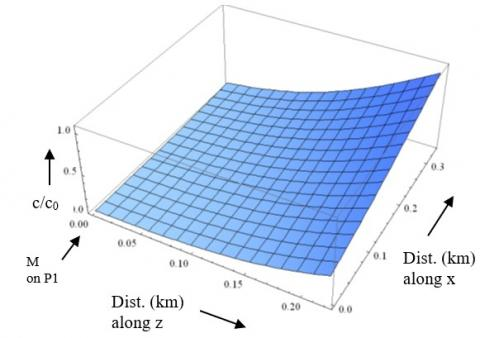Figure 11. Surface plot of concentration obtained with Eq. (34) for plane source at time t(year)=0.08 in xz plane

4. Conclusion

The analytical solutions, of three-dimensional advection-dispersion equation (ADE) in an infinite region of aquifer bounded between two infinite parallel planes, are evaluated by using Laplace Transformation Technique. Source of the pollutant entered through left plane in case of along the flow while in against the same is entering through right plane as shown in Figure 1(a) and Figure 1(b). Laplace transformation technique has been used to get the analytical solutions.  The variable coefficients of the advection–diffusion equation are converted into constant coefficients with the of new independent variables introducing through different transformations. A new independent variable introduced through the transformation in equation (6) serves as a new time variable. Though the analytical solutions have illustrated for $f(m t)=\exp (-m t)$ i.e. exponentially decreasing function of time but the result may also hold for linear functions, quadratic or sinusoidal expressions. It is found that the contaminant concentration decreases as distance increases from the source plane in both the directions i.e., along and against the flow and achieves slowly to its lowest level at another non-source infinite plane. For both type of flow i.e., along and against the solutions are obtained for line and point sources in one-and two-dimensional ADE respectively.  The result may be helpful to compare the contaminant level at any position and time of transport when source is applied in the direction and against the flow.

Nomenclature

c       concentration of the solute, kg m-3

Dxx   components of dispersion coefficient along x axis, m2s-1

Dyy    components of dispersion coefficient along y axis, m2s-1

Dzz    components of dispersion coefficient along z axis, m2s-1

ux     components of groundwater velocity along x axis, ms-1

uy     components of groundwater velocity along y axis, ms-1

uxz     components of groundwater velocity along z axis, ms-1

Dxx0  initial dispersion coefficient along x axis, m2s-1,

Dyy0  initial dispersion coefficient along y axis, m2s-1

Dzz initial dispersion coefficient along z axis, m2s-1

ux0    initial groundwater velocity along x axis, ms-1

uy0    initial groundwater velocity along y axis, ms-1

uz0    initial groundwater velocity along z axis, ms-1

c0     reference / source concentration

ci       initial concentration

distance measured x axis, m

y      distance measured y axis, m

distance measured z axis, m

time, s

m     unsteady parameter regulates dispersion and groundwater velocity, s-1

R     dimensionless retardation factor

$a_{1}, b_{1}, c_{1}$,$f_{1}, f_{2}$    constants those determine the planes

References

 Fried, J.J. (1972). Miscible pollution of groundwater: A study of methodology. In Proceedings of the International Symposium on Modelling Techniques in Water Resources Systems, vol. 2, Ottawa, Canada, pp. 362-371.

 Rumer, R.R. (1962). Longitudinal dispersion in steady and unsteady flow. Journal of the Hydraulics Division, 88(HY4): 147-172.

 Matheron, G., De Marsily, G. (1980). Is transport in porous media always diffusive? A counterexample. Water Resources Research, 16(5): 901-917. https://doi.org/10.1029/WR016i005p00901

 Huang, K., Van Genuchten, M.T., Zhang, R. (1996). Exact solutions for one-dimensional transport with asymptotic scale-dependent dispersion. Applied Mathematical Modelling, 20: 298-308.

 Singh, M.K., Das, P., Singh, V.P. (2015). Solute transport in a semi-infinite geological formation with variable porosity. J. Engineering Mechanics, ASCE, 141(11). http://doi.org/10.1061/(ASCE)EM.1943-7889.0000948

  Logan, J.D. (1996). Solute transport in porous media with scale dependent dispersion and periodic boundary conditions. J. Hydrol, 184(3-4): 261-276.

 Zlotnik, V., Ledder, G. (1996), Theory of dipole flow in uniform anisotropic aquifers. Water Resource Research, 32(4): 1119-1128. http://doi.org/10.1029/95WR03813

 Ellsworth, T.R., Butters, G.L. (1993). Three-dimensional analytical solutions to the advection-dispersion equation in arbitrary cartesian coordinates. Water Resource Research, 29(9): 3215-3225. http://doi.org/10.1029/93WR01293

 Batu, V. (1989). A generalized two-dimensional analytical solution for hydrodynamic dispersion in bounded media with the first-type boundary condition at the source. Water Resources Research, 25(6): 1125-1132. http://doi.org/10.1029/WR025i006p01125

 Aral, M.M., Liao, B. (1996). Analytical solutions for two-dimensional transport equation with time dependent dispersion coefficients. Journal of Hydrologic Engineering, 1(1): 20-32. http://doi.org/10.1061/(ASCE)1084-0699(1996)1:1(20)

 Zoppou, C., Knight, J.H. (1997). Analytical solution for advection and advection-diffusion equations with spatially variable coefficients. J. Hydraul. Eng., ASCE, 123(2): 144-148. http://doi.org/10.1061/(ASCE)0733-9429(1997)123:2(144)

 Van Genuchten, M.T., Leij, F.J., Skaggs, T.H., Toride, N., Bradford, S.A., Pontedeiro E.M. (2013). Exact analytical solutions for contaminant transport in rivers 1. The equilibrium advection dispersion equation. J. Hydrol. Hydromech, 61(2): 146-160. http://doi.org/10.2478/johh-2013-0020

 Mahato, N.K., Begam, S., Das, P., Singh, M.K. (2015). Two-dimensional solute dispersion along and against the unsteady groundwater flow in aquifer. Journal of Groundwater Research, Vol.3, 4/1.

 Batu, V. (1996). A generalized three-dimensional analytic solute transport model for multiple rectangular first type sources. J. Hydrol., 174(1-2): 57-82. http://doi.org/10.1016/0022-1694(95)02752-1

 Park, E., Zhan, H. (2001). Analytical solutions of contaminant transport from finite one-, two-, and three-dimensional sources in a finite-thickness aquifer. J. Cont. Hydrol., 53: 41-61. http://doi.org/10.1016/S0169-7722(01)00136-X

 Zamani, K., Bombardelli, F.A. (2014). Analytical solutions of nonlinear and variable-parameter transport equations for verifications of numerical solvers. Environ. Fluid Mech., 14(4): 711-742. http://doi.org/10.1007/s10652-013-9325-0

 Kumar, A., Kumar, L.K., Shireen (2018). Analysis of two-dimensional solute transport through heterogeneous porous medium. Journal of Applied Mathematics and Computation, 2(3): 67-83. http://dx.doi.org/10.26855/jamc.2018.03.001

 Yadav, R.R., Roy, J. (2019). Solute transport in a semi-infinite porous media with input through a curve line source. International Journal of Advances in Mathematics, 2019(4): 35-52.

 Thakur, C.K., Chaudhary, M., van der Zee, S.E.A.T.M., Singh, M.K. (2019). Two-dimensional solute transport with exponential initial concentration distribution and varying flow velocity. Pollution, 5(4): 721-737. http://dx.doi.org/10.22059/poll.2019.275005.574

 Pandey, A.K., Kumar, R., Singh, M.K. (2018). Solution to advection–dispersion equation for the heterogeneous medium using Duhamel’s principle. Applications of Fluid Dynamics, pp. 559-571. http://doi.org/10.1007/978-981-10-5329-0_42

 Singh, M.K., Chatterjee, A. (2016). Solute dispersion in a semi-infinite aquifer with specified concentration along an arbitrary plane source. Journal of Hydrology, 541: 928-934. http://doi.org/10.1016/j.jhydrol.2016.08.003

 Bear, J. (1972). Dynamics of Fluids in Porous Media. American Elsevier, New York.

 Yadav, R.R., Jaiswal, D.K., Yadav, D.K., Gulrana (2012). Three-dimensional temporally dependent dispersion through porous media: An analytical solution. Environ Earth Sci., 65: 849-859. http://doi.org/10.1007/s12665-011-1129-2

 Bear, J., Verruijt, A. (1984). Modelling Groundwater Flow and Pollution, D. Reidel Publishing Company, Holland.

 Jaiswal, D.K., Gulrana, Yadav, R.R. (2013), Solute-transport under fluctuating groundwater flow in homogeneous finite porous domain. J Hydrogeol Hydrol Eng., 2: 1. http://doi.org/10.4172/2325-9647.1000103

 Crank, J. (1975). The Mathematics of Diffusion, (2ndedn). Oxford Univ Press Inc., New York, USA.

 Yadav, R.R., Jaiswal, D.K., Gulrana. (2011). Analytical solution for solute transport in one-dimensional porous media with a periodic boundary condition. International Journal of Pure and Applied Mathematical Sciences, 5(1-2): 1-13.

 Yadav, R.R., Roy, J., Jaiswal, D.K. (2019). One-dimensional solute transport for an input against the flow in a homogeneous finite porous media. International Journal of Engineering Technologies, 5(2).

 Todd, D.K., (1980). Groundwater Hydrology. John Wiley, New York, USA.